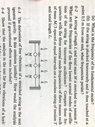# Normal modes of oscillation

• Jack-Dylan96
I suggest looking at the homework help on this site which will walk you through the process step-by-step.Thanks for your help in this.

#### Jack-Dylan96

Homework Statement
A string of length L and total mass M is stretched to a tension T. What are the frequencies of the three lowest normal modes of oscillation of the string for transverse oscillations. Compare these frequencies with the three normal mode frequencies of three masses each of mass M/3 spaced at equal intervals on a massless string of tension T and total length L.
Relevant Equations
v_n=(n/2L)*(TL/M)^(1/2)
The first part is trivial not sure where to go on the second part.

Jack-Dylan96 said:
Homework Statement:: A string of length L and total mass M is stretched to a tension T. What are the frequencies of the three lowest normal modes of oscillation of the string for transverse oscillations. Compare these frequencies with the three normal mode frequencies of three masses each of mass M/3 spaced at equal intervals on a massless string of tension T and total length L.
Relevant Equations:: v_n=(n/2L)*(TL/M)^(1/2)

The first part is trivial not sure where to go on the second part.
Welcome to PF.

Please show your work on the trivial part so we can see how you work this problem. Then mention one or two ways that you could maybe approach the second part. We cannot provide tutorial help until you show your work. Thanks.

What are the equations of motion for each mass?

The first part is simply a case of invoking the formula for normal mode frequencies and plugging in integers 1,2,3 giving.

V_1=(1/2L)*(TL/M)^(1/2) and V_2=(1/L)*(TL/M)^(1/2) hence nv_1 (n=1,2,3) second part I assume is a case of dividing the string up into parts as per the attached photo but cannot see how to derive the correct solution of 0.84v_1, 1.55v_1 and 2.54v_1

#### Attachments

•image.jpg
63.2 KB · Views: 67
So what are the forces acting on each mass as a function of the transversal displacement of the masses?

I am unsure are you able to provide any assistance on this. The force will likely involve the tension in the string and be a function of the angle at which the string makes with the horizontal.

Jack-Dylan96 said:
I am unsure are you able to provide any assistance on this.
With this attitude I think you need to take a look at the homework guidelines of Physics Forums. We will help you and guide you. We will not solve the problem for you.

Jack-Dylan96 said:
The force will likely involve the tension in the string and be a function of the angle at which the string makes with the horizontal.
So, look at mass 1. What are the vertical forces acting on it in terms of the tension and the angles of the strings? Can you find a way to express the angles using the positions of the masses?

Thanks for your help in this.

Jack-Dylan96 said:
I am unsure are you able to provide any assistance on this. The force will likely involve the tension in the string and be a function of the angle at which the string makes with the horizontal.
If @Orodruin is unable to help you, it is not for lack of knowledge on his part.

I’m not suggesting anything of the sort, I feel I may have posted this in the wrong place given my predicament. And I do appreciate the help and time you have given me, thank you.

Jack-Dylan96 said:
I’m not suggesting anything of the sort, I feel I may have posted this in the wrong place given my predicament. And I do appreciate the help and time you have given me, thank you.
Physics Forums is different than many Q&A sites on the internet. We expect students and others posting in the homework forums to both have made and show their efforts as well as be willing to work with our hints and guidance towards the solution. This is generally a process over several posts unless it is just pointing out some minor mistake. Posting your work let's us assess how far you have cone and exactly where you are stuck rather than having us guess and leading you from the beginning. This is most often a better approach to learning than simply telling you the answer. You will discover aspects as independently as possible meaning that they will also stick better.

Your ideas about the forces on the masses are good, but you need to start working with them and converting them to math so we can understand where you really get stuck.

•kuruman and SammyS